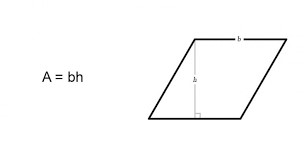# Haskell Program to Find the Area of a parallelogram

This tutorial discusses writing a program to print the area of a parallelogram in the Haskell programming language.

A parallelogram is a quadrilateral with opposite sides that have the same size and are parallel to each other.In this tutorial, We see three ways to implement

• Program to compute the area of a parallelogram using height and base.
• Program to compute the area of a parallelogram using sides.
• Program to compute the area of a parallelogram using the diagonals.

## Algorithm Steps

• Take input or initialize the variable for the size of the parallelogram.
• Implement the program logic to compute the area of the parallelogram.
• Print or display the area.

## Example 1

Program to compute the area of a parallelogram using height and base.

main :: IO()
main = do
-- declaring and initializing the variable for base and height
let base = 5
let height = 6
-- computing area
let area = base * height
-- printing the area
print ("Area of the parallelogram with base "++ show base ++ " and height " ++ show height ++ " is:")
print (area)


## Output

"Area of the parallelogram with base 5 and height 6 is:"
30


Note − The function show parses numbers into a string. It takes a number as an argument and returns the parsed string. “++” is an operator to concatenate strings.

In the above program, we declared and initialized variables for the base and height. The area of the parallelogram with a base and height can be computed by base*height. We computed the area by multiplying the base with height and loading it into a variable area. Finally, we printed the area of the parallelogram.

## Example 2

Program to compute the area of a parallelogram using sides.

main :: IO()
main = do
-- declaring and initializing the variable for sides and angle
let a = 5
let b = 6
let angle = pi/4
-- computing area
let area = a*b*(sin angle)
-- printing the area
print ("Area of the parallelogram with sides "++ show a ++ " " ++ show b ++ " and angle between them " ++ show angle)
print (area)


## Output

"Area of the parallelogram with sides 5.0 6.0 and angle between them 0.7853"
21.213203435596423


In the above program, we declared and initialized the variables for the sides and angles between them. The area of the parallelogram with sides of lengths a and b and the angle between them is k, then the area is a*b*sin(k). We computed the area and loaded it into a variable area. Finally, we printed the area of the parallelogram.

## Example 3

Program to compute the area of a parallelogram using the Diagonals.

main :: IO()
main = do
-- declaring and initializing the variable for base and height
let d1 = 6
let d2 = 8
let angle = pi/3
-- computing area
let area = (0.5)*d1*d2*(sin angle)
-- printing the area
print ("Area of the parallelogram with diagonals "++ show d1 ++ " " ++ show d2 ++ " and angle between them " ++ show angle)
print (area)


## Output

"Area of the parallelogram with diagonals 6.0 8.0 and angle between them 1.047197"
20.784609690826528


In the above program, we declared and initialized variables for diagonals and angle between them. The area of the parallelogram with diagonals d1,d2, and the angle between them k is given by ½*d1*d2*sin(k). We computed the area and loaded it into a variable area. Finally, we printed the area.

## Conclusion

In this tutorial, we discussed three ways to implement the program to compute the area of the parallelogram in the Haskell programming language.

Updated on: 14-Dec-2022

147 Views